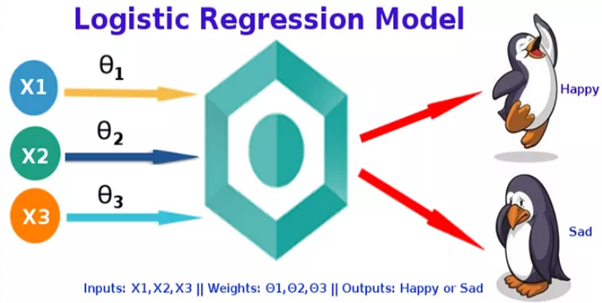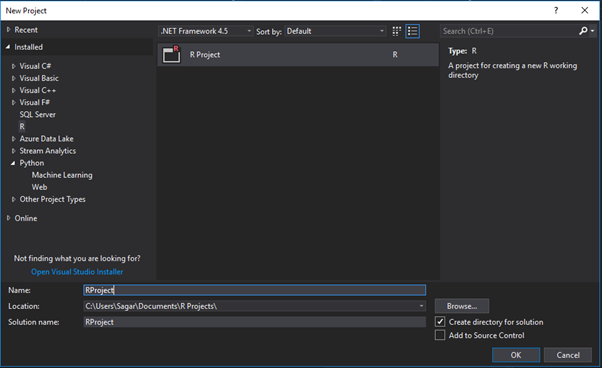# Data Science Training in Mumbai : – Using Logistic Regression with R Programming using Visual Studio.The Logistic Regression is special type regression model in which the response variable also known as dependent variable has categorical values such as 0/1 or True/False.

Logistic Regression in reality measures the probability of a binary response as the value of response variable based on the mathematical equation relating it with the predictor variables.

Cases where the dependent variable has more than two outcome categories may be analysed in multinomial logistic regression, or, if the multiple categories are ordered, in ordinal logistic regression.

The binary response can be estimated using binary logistic model on one or more predictor or independent variables aka features.

It lets one to say that the presence of this risk factor will increase the odds of a given outcome by a specific factor and the model is not a classifier but a direct probability model.

The equation for Logistic Regression is as can be used follows:

y = 1/(1+e^-(a+b1x1+b2x2+b3x3+…))

Following is the description of the parameters used −

• y :It is a response variable.
• x :It is a predictor variable.
• a , b :They are the coefficients which represent numeric constants.

The glm() function is used to create regression model.

Syntax

The glm() function is used for a general equation for logistic regression is −

glm(formula,data,family)

Where

• formula :It is the symbol presenting the relationship between the variables.
• data :It is the dataset which gives the values of these variables.
• family :It is R object to specify the details of the model and it has a value which is binomial for logistic regression.

To create and run our R program in Visual Studio we must first install some tools.

Now open Visual Studio on your pc and then click on Get Tools and features… inside Tools.

Now in the feature installation window check and install the following features.

For Visual Studio 2017

Contains Tools for Data Science

After this please install R tools from the following link if the above package is not installed properly from the following link

But it is not necessary

https://docs.microsoft.com/en-us/visualstudio/rtvs/installing-r-tools-for-visual-studio

You can also install R programming tool go to the following link for better support and download the software and install it

For Windows Users:

https://ftp.iitm.ac.in/cran/

For Linux Users:

If you are on Linux platform then you can use this fast and easy command used in Linux which can be used to install R. The yum command is used for installing like this:

\$ yum install R

For Ubuntu Linux or other Debian-related OSs, a more direct method is:

\$ apt-get install r-base

Now let us start creating our R application in visual studio

Go to Files ->Project and add a new R projectThis is our project structure:

Now on the top left side is a new R file (script.R) where we can edit source code with all of Visual Studio Ide editing features.

Also on the bottom left of Visual Studio is where you can find an R Interactive window in which you can interactively develop and test code.

We can directly use R Interactive without the need of opening any new project.

Now let us see a demonstration of Logistic regression:

The pre-existing dataset “mtcars” describes different models of a car with their various engine specifications.

In “mtcars” data set transmission mode (automatic or manual) is described by the column am which a binary value (0 or 1) is.

Here we can create a logistic regression model between the different columns namely “am” and 3 other columns – hp, cyl and wt.

input <- mtcars[,c("am","cyl","hp","wt")]

# applying Logistic regression to the data
am.data = glm(formula = am ~ cyl + hp + wt, data = input, family = binomial)
print(am.data)
print(summary(am.data))

Output:

Syllabus of Data Science training in Mumbai